## Screenshots

•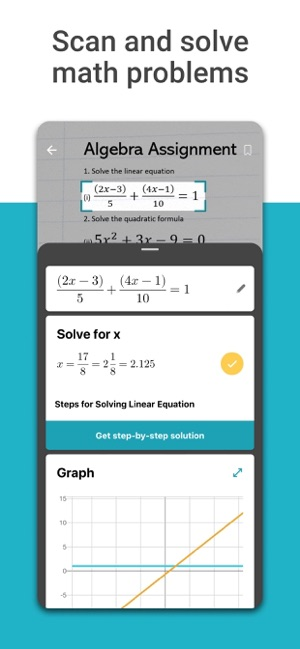•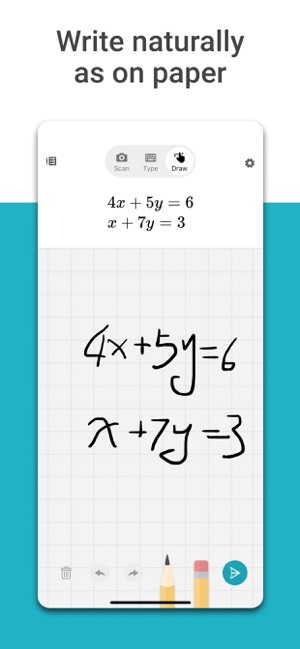•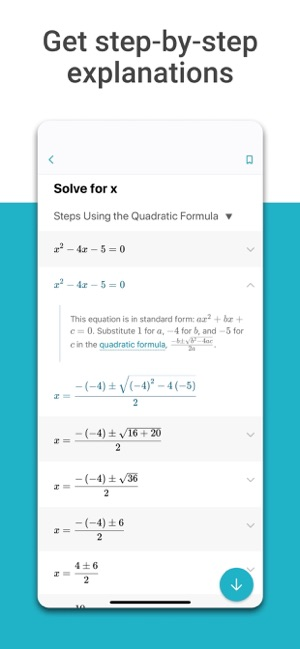•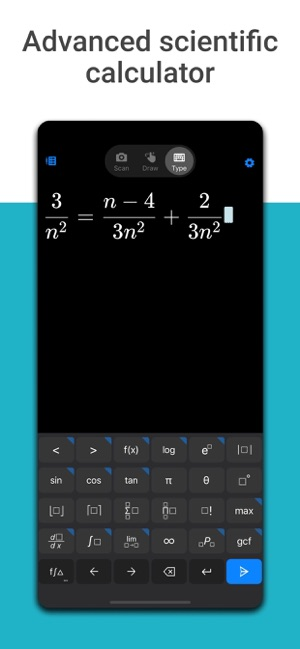•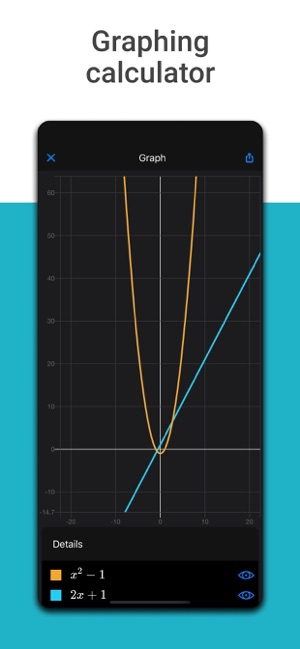•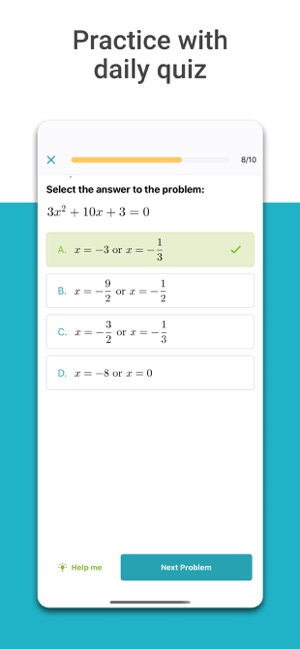•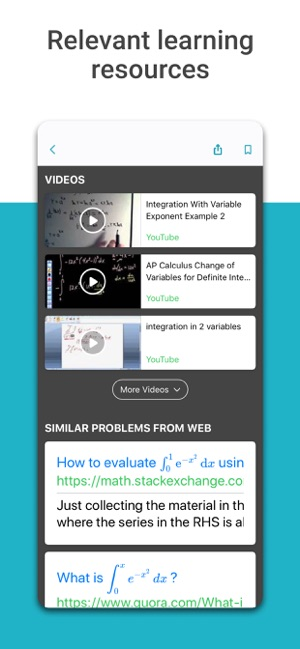## Description

Microsoft Maths solver app provides help with a variety of problems including arithmetic, algebra, trigonometry, calculus, statistics, and other topics using an advanced AI powered maths solver. Simply write a maths problem on screen or use the camera to snap a maths photo. Microsoft Maths instantly recognizes the problem and helps you to solve it with step-by-step explanation, interactive graphs, similar problems from the web and online video lectures. Quickly look up related maths concepts. Get help with your homework problems and gain confidence in mastering the techniques with Microsoft Mathematics. It is absolutely FREE!

● Write a maths equation on screen as you naturally do on paper
● Scan printed or handwritten maths photo
● Type and edit using advanced scientific maths calculator
● Get interactive Step-by-Step explanation & Graphing calculator
● Import images with maths equations from gallery
● Scan and Solve Maths Worksheets with multiple problems
● Search the web for similar problems and video lectures
● Try maths word problems
● Scan and plot x-y data tables for linear/non-linear functions
● Learn maths in your language - supports Chinese, French, German, Hindi, Italian, Japanese, Portuguese, Russian, Spanish, and many more

SUPPORTED PROBLEMS
● Elementary: arithmetic, real, complex numbers, LCM, GCD, factors, roman numerals
● Pre-Algebra: radicals and exponents, fractions, matrices, determinants
● Algebra: quadratic equations, system of equations, inequalities, rational expressions, linear, quadratic and exponential graphs
● Word problems on maths concepts, number theory, probability, volume, surface area
● Basic Calculus: Summations, Limits, derivatives, integrals
● Statistics: Mean, Median, Mode, Standard Deviation, permutations, combinations

Microsoft Maths solver combines the power of multiple solvers such as maths problem solver, equation solver, maths answer scanner, percentage calculator, scientific calculator, word problem solver, maths photo solver and maths handwriting solver. This is a powerful maths learner app for solving maths homework problems. Find out more about Microsoft Maths Solver app on our website: https://math.microsoft.com

## What’s New

Version 1.0.15

- Daily practice quiz, personalized to the problems you are interested in.
- Performance improvements and bug fixes

4.7 out of 5
30 Ratings

30 Ratings

## Information

Provider
Microsoft Corporation
Size
34.6 MB
Category
Education
Compatibility

Requires iOS 11.0 or later. Compatible with iPhone, iPad and iPod touch.

Languages

English, Arabic, Chinese, Czech, Dutch, French, German, Greek, Hebrew, Hindi, Hungarian, Indonesian, Italian, Japanese, Korean, Malay, Polish, Portuguese, Romanian, Russian, Simplified Chinese, Slovak, Spanish, Thai, Traditional Chinese, Turkish, Vietnamese

Age Rating
4+
Price
Free

## Supports

•